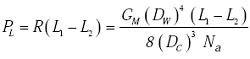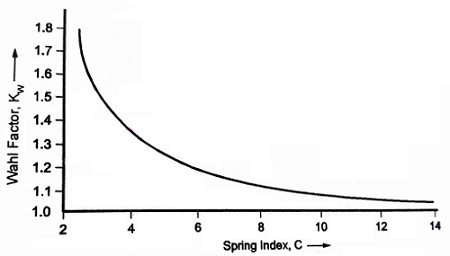Related Resources: calculators

### Compression Spring Assembly Operating Life and Reliability Equations and Calculator

Spring Engineering and Design

Compression Spring Assembly Operating Life and Reliability Equations and Calculator

Depending on the application, a spring may be in a static, cyclic, or dynamic operating mode. In the current example of a valve assembly, the spring will be in a cyclic mode. The operating life of a mechanical spring arrangement is dependent upon the susceptibility of the materials to corrosion and stress levels (static, cyclic or dynamic). The most common failure modes for springs include fracture due to fatigue and excessive loss of load due to stress relaxation. Other failure mechanisms and causes may be identified for a specific application. Typical failure rate considerations include: level of loading, operating temperature, cycling rate and corrosiveness of the fluid environment.

The failure rate of a compression spring depends upon the stress on the spring and the relaxation properties of the material. The load on the spring is equal to the spring rate multiplied by the deflection and calculated:Where:

R = Spring rate, lb/in
L1 = Initial deflection of spring, in
L2 = Final deflection of spring, in
GM = Modulus of rigidity, lb/in2
DW = Mean diameter of wire, in
DC = Mean diameter of spring, in
Na = Number of active coils

Stress in the spring will be proportional to loading according to the following relationship:Where:

SG = Actual stress, psi
KW = Wahl stress correction factorThis equation permits determination of expected life of the spring by plotting the material S-N curve on a modified Goodman diagram. In the example valve application, the spring force and the failure rate remain constant. This projection is valid if the spring does not encounter temperature extremes. Corrosion is a critical factor in spring design because most springs are made of steel which is susceptible to a corrosive environment. In this example the fluid medium is assumed to be non-corrosive and the spring is always surrounded by the fluid, thus a corrosion factor need not be included in this analysis. If the valve were a safety device and subjected intermittently to a steam environment, then a corrosion factor would have to be applied consistent with any corrosion protection in the original spring design.

The failure rate of the compression spring can be estimated from the following equation:where:

TS = Material tensile strength, lbs/in2
λSP,B = Base failure rate for spring assembly, failures/million cycles

References:

Handbook of Reliability Prediction Procedures for Machinal Equipment

Related: